## YOLO2解读，训练自己的数据及相关转载以供学习

https://pjreddie.com/darknet/yolo/ 具体安装及使用可以参考官方文档
https://github.com/pjreddie/darknet

http://blog.csdn.net/u012235274/article/details/52399425 caffe-yolo 训练
http://blog.csdn.net/u012235274/article/details/52120152 caffe 版本 yolo 过程记录

YOLOv2 参数详解
[net]
// http://guanghan.info/blog/en/my-works/train-yolo/
// if you have 64 images as a batch, for example, you batch update the weights upon processing 64 images.
batch=64
// if you have subdivision to be set to 8, you have 8 images for each subdivision. For each division, you concatenate the ground truth image feature vectors into one and process it as a whole.
// so If you set subdivision to 2, the training is the fastest, but you see less results printed out.
subdivisions=8
width=416
height=416
channels=3
momentum=0.9
decay=0.0005
// 对于每次迭代训练，YOLOv2会基于角度(angle)，饱和度(saturation)，曝光(exposure)，色调(hue)产生新的训练图片
// angle：图片角度变化，单位为度，假如angle=5，就是生成新图片的时候随机旋转-5~5度
// saturation & exposure: 饱和度与曝光变化大小，1.5~1倍
// hue：色调变化范围，-0.1~0.1
angle=0
saturation = 1.5
exposure = 1.5
hue=.1

learning_rate=0.001 // 初始化学习率
max_batches = 40100 //训练达到max_batches后停止学习
policy=steps //按照steps策略调整学习率，还有EXP，CONSTANT，POLY等
steps=-1,100,20000,30000 //根据batch_num调整学习率，
scales=.1,10,.1,.1 //学习率变化的比例，累计相乘

[region]
anchors = 1.08,1.19, 3.42,4.41, 6.63,11.38, 9.42,5.11, 16.62,10.52
bias_match=1
classes=20
coords=4 //BoundingBox的tx,ty,tw,th，tx与ty是相对于左上角的gird，同时是当前grid的比例，tw与th是宽度与高度取对数
num=5 // 每个grid预测的BoundingBox个数
softmax=1 //使用softmax作为激活函数
jitter=.2 //通过抖动增加噪声，控制过拟合
rescore=1 //理解为一个开关，非零时通过重新打分来调整I.delta（预测值与真实值的差）

//YOLOv1论文中cost function的权重，哪一个更大，每一次更新权重的时候，对应方面的权重更新相对比重更大
object_scale=5 // 计算损失时，预测框中有物体的权重
noobject_scale=1 // 计算损失时，预测框中没有物体的权重
class_scale=1 // 计算类别损失时的权重
coord_scale=1 // 计算损失时坐标偏差的权重

absolute=1
thresh = .6 //决定是否需要计算IOU误差的参数，大于thresh，IOU误差不会夹在cost function中
random=1 //是否随机确定最后一个预测框

http://blog.csdn.net/q6324266/article/details/54375452 darknet yolo v2 训练自己的数据
http://blog.csdn.net/hysteric314/article/details/54097845 【YOLO学习】使用YOLO v2训练自己的数据
http://blog.csdn.net/burning_keyboard/article/details/71056164 yolo训练自己的数据实践总结

http://blog.csdn.net/ch_liu23/article/details/53558549

1.修改 cfg/voc.data：
classes= 10
train = path/to/trainImagePath.txt
validate = path/to/validateImagePath.txt
names = data/classes.names
backup = backup // 记录每迭代100次得到的模型 .weights文件

2.修改网络结构 cfg/yolo-voc.cfg：
（1）最后一个卷积层
[convolutional]
size=1
stride=1
filters=125 // 125 = 5(anchor box) * (20(class) + 4(coor) + 1(confidence))
... // 根据class数算好filters个数
（2）[region]
anchors = 1.08,1.19, 3.42,4.41, 6.63,11.38, 9.42,5.11, 16.62,10.52
bias_match=1
classes=10 // 改成目标分类数量
coords=4
num=5 // anchor box 数量
...
（3）预训练文件cfg/darknet19_448.conv.23：在其他数据集上pretrain的模型做为初值
3.训练命令
cd darknet
./darknet detector train cfg/voc.data cfg/yolo-voc.cfg cfg/darknet19_448.conv.23

4.检测命令
./darknet detector test cfg/voc.data cfg/yolo-voc.cfg backup/yolo-voc_400.weights testImage/738780.jpg

https://github.com/hgpvision/darknet

darknet 中最重要的三个struct定义是 network_state, network, layer；

train_network_datum 顺序执行 forward_network { 逐层正向网络 } backward_network { 逐层逆向网络 }，

policy 是weights 策略的， inputs batch(及相关的subdivisions) ouputs 是与数据吞吐维度相关的，最新版本好像ouputs这里有改正。

https://zhuanlan.zhihu.com/p/25167153 YOLO2详解答
http://blog.csdn.net/jesse_mx/article/details/53925356

## 回顾YOLOv1

YOLOv2始终是在v1版本上作出的改进，我们先简单回顾YOLOv1的检测步骤：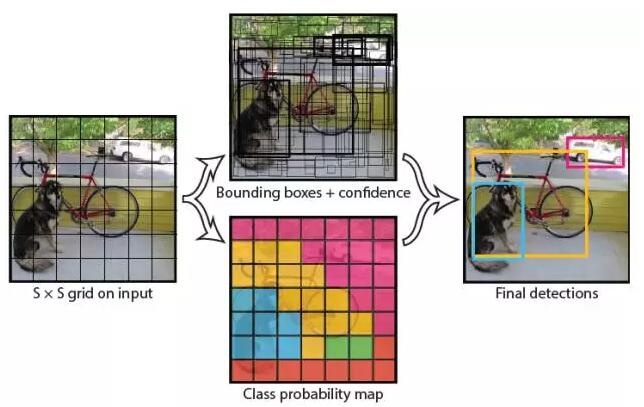(1) 给个一个输入图像，首先将图像划分成7 * 7的网格。

(2) 对于每个网格，每个网格预测2个bouding box（每个box包含5个预测量）以及20个类别概率，总共输出7×7×（2*5+20）=1470个tensor

(3) 根据上一步可以预测出7 * 7 * 2 = 98个目标窗口，然后根据阈值去除可能性比较低的目标窗口，再由NMS去除冗余窗口即可。

YOLOv1使用了end-to-end的回归方法，没有region proposal步骤，直接回归便完成了位置和类别的判定。种种原因使得YOLOv1在目标定位上不那么精准，直接导致YOLO的检测精度并不是很高。

YOLO检测原理参考推荐博客：论文阅读：You Only Look Once: Unified, Real-Time Object Detection

## YOLOv2精度的改进（Better）### Batch Normalization

CNN在训练过程中网络每层输入的分布一直在改变, 会使训练过程难度加大，但可以通过normalize每层的输入解决这个问题。新的YOLO网络在每一个卷积层后添加batch normalization，通过这一方法，mAP获得了2%的提升。batch normalization 也有助于规范化模型，可以在舍弃dropout优化后依然不会过拟合。

### Convolutional With Anchor Boxes### Dimension Clusters（维度聚类）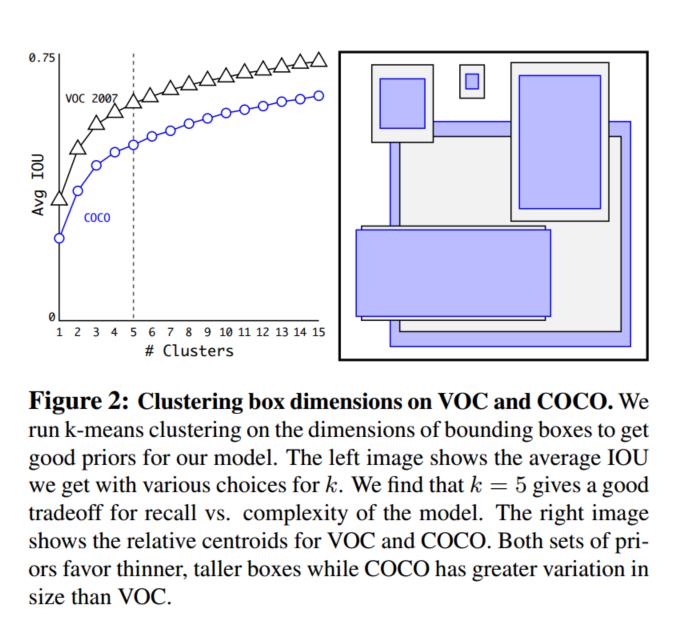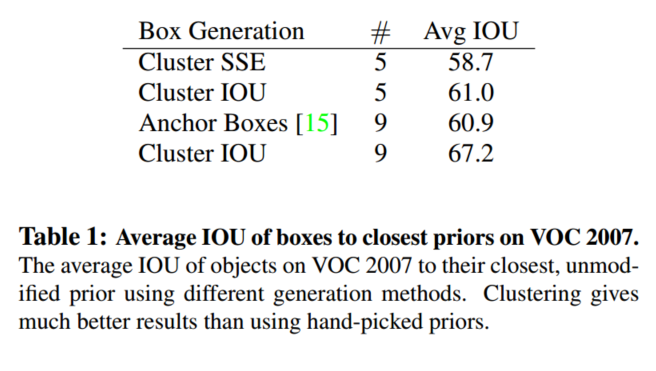### Direct location prediction（直接位置预测）x=(txwa)+xay=(tywa)+ya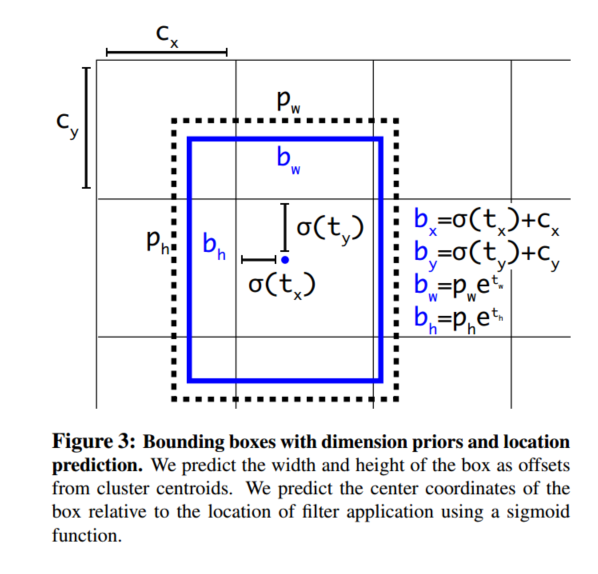### Fine-Grained Features（细粒度特征）### Multi-Scale Training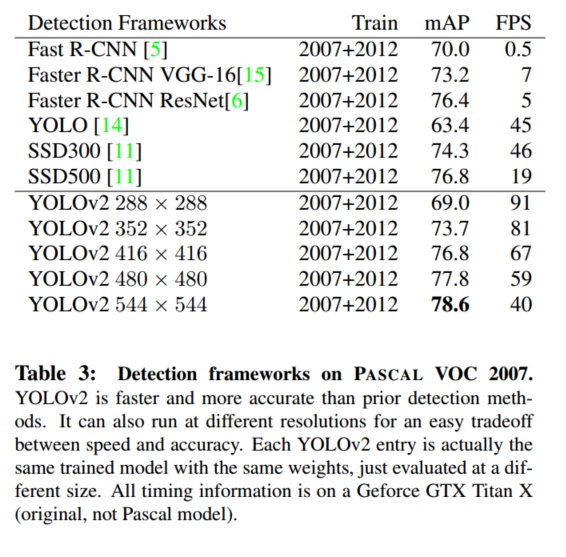### Further Experiments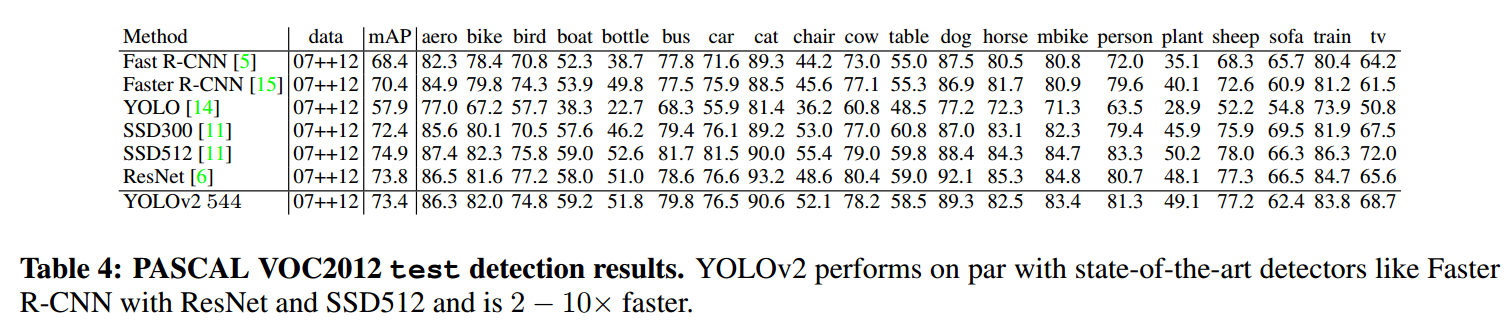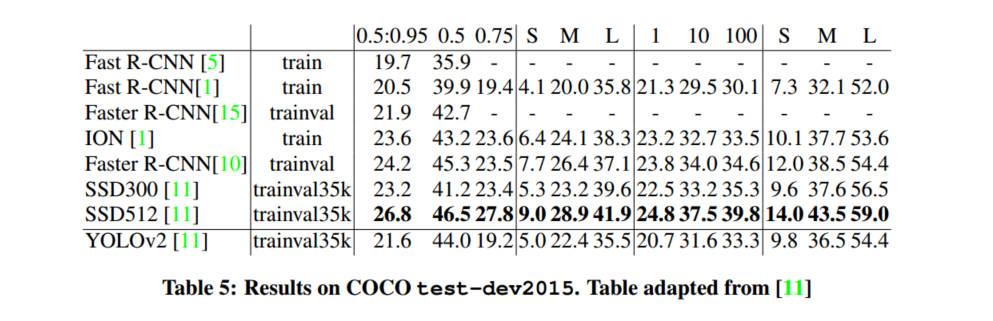## YOLOv2速度的改进（Faster）

YOLO一向是速度和精度并重，作者为了改善检测速度，也作了一些相关工作。

### Darknet-19

YOLOv2使用了一个新的分类网络作为特征提取部分，参考了前人的先进经验，比如类似于VGG，作者使用了较多的3 * 3卷积核，在每一次池化操作后把通道数翻倍。借鉴了network in network的思想，网络使用了全局平均池化（global average pooling），把1 * 1的卷积核置于3 * 3的卷积核之间，用来压缩特征。也用了batch normalization（前面介绍过）稳定模型训练。## 总结和展望

region_layer.c
box get_region_box(float *x, float *biases, int n, int index, int i, int j, int w, int h, int stride)
{
box b;
b.x = (i + x[index + 0*stride]) / w;
b.y = (j + x[index + 1*stride]) / h;
b.w = exp(x[index + 2*stride]) * biases[2*n]   / w;
b.h = exp(x[index + 3*stride]) * biases[2*n+1] / h;
//printf("%f/%d/%d - %f/%f/%f/%f\n", x[index + 2*stride], w, h, b.x, b.y, b.w, b.h);
return b;
}

float delta_region_box(box truth, float *x, float *biases, int n, int index, int i, int j, int w, int h, float *delta, float scale, int stride)
{
box pred = get_region_box(x, biases, n, index, i, j, w, h, stride);
float iou = box_iou(pred, truth);

float tx = (truth.x*w - i);
float ty = (truth.y*h - j);
float tw = log(truth.w*w / biases[2*n]);
float th = log(truth.h*h / biases[2*n + 1]);

delta[index + 0*stride] = scale * (tx - x[index + 0*stride]);
delta[index + 1*stride] = scale * (ty - x[index + 1*stride]);
delta[index + 2*stride] = scale * (tw - x[index + 2*stride]);
delta[index + 3*stride] = scale * (th - x[index + 3*stride]);
return iou;
}

void forward_region_layer()
{
...

for (b = 0; b < l.batch; ++b) {
if(l.softmax_tree){
// 没执行
}
// 下面的 for 循环是计算没有物体的 box 的 confidence 的 loss
// 1， 遍历所有格子以及每个格子的 box，计算每个 box 与真实 box 的 best_iou
// 2， 先不管三七二十一，把该 box 当成没有目标来算 confidence 的 loss
// 3， 如果当前 box 的 best_iou > 阈值，则说明该 box 是有物体的，于是上面哪行计算的 loss 就不算数，因此把刚才计算的 confidence 的 loss 清零。
// 假设图片被分成了 13 * 13 个格子，那 l.h 和 l.w 就为 13
// 于是要遍历所有的格子，因此下面就要循环 13 * 13 次
for (j = 0; j < l.h; ++j) {
for (i = 0; i < l.w; ++i) {
// 每个格子会预测 5 个 boxes，因此这里要循环 5 次
for (n = 0; n < l.n; ++n) {
// 获得 box 的 index
int box_index = entry_index(l, b, n*l.w*l.h + j*l.w + i, 0);
// 获得 box 的预测 x, y, w, h，注意都是相对值，不是真实坐标
box pred = get_region_box(l.output, l.biases, n, box_index, i, j, l.w, l.h, l.w*l.h);
float best_iou = 0;
// 下面的循环 30 次我是这么理解的：
//        假设一张图片中最多包含 30 个物体，于是对每一个物体求 iou
// PS：我看了很久都没找到这个 30 能和什么关联上，于是猜测 30 的含义是“假设一张图片中最多包含 30 个物体”。
for(t = 0; t < 30; ++t){
// get truth_box's x, y, w, h
box truth = float_to_box(net.truth + t*5 + b*l.truths, 1);
printf("\ti=%d, j=%d, n=%d, t=%d\n", i, j, n, t);
// 遍历完图片中的所有物体后退出
if(!truth.x){
break;
}
float iou = box_iou(pred, truth);
if (iou > best_iou) {
best_iou = iou;
}
}
// 获得预测结果中保存 confidence 的 index
int obj_index = entry_index(l, b, n*l.w*l.h + j*l.w + i, 4);
avg_anyobj += l.output[obj_index];
// 这里先不管三七二十一，直接把该 box 当成没有目标来算 loss 了。
l.delta[obj_index] = l.noobject_scale * (0 - l.output[obj_index]);
// 然后再做个判断，如果当期 box 计算的 best_iou > 阈值的话，则说明该 box 是有物体的，于是上面哪行计算的 loss 就不算数，因此清零。
if (best_iou > l.thresh) {
l.delta[obj_index] = 0;
}

// 查了查代码，这里是“如果已经训练的图片数量 < 12800 的话则进入循环”，为什么要判断这玩意....
if(*(net.seen) < 12800){
// 单纯的获取“以当前格子中心”为 x, y 的 box 作为 truth box
box truth = {0};
truth.x = (i + .5)/l.w;
truth.y = (j + .5)/l.h;
truth.w = l.biases[2*n]/l.w;
truth.h = l.biases[2*n+1]/l.h;
// 将预测的 tx, ty, tw, th 和 实际box计算得出的 tx',ty', tw', th' 的差存入 l.delta
delta_region_box(truth, l.output, l.biases, n, box_index, i, j, l.w, l.h, l.delta, .01, l.w*l.h);
}
}
}
}
// 下面的循环 30 次中的 30 这个数我看了很久都没找到这个 30 能和什么关联上，于是猜测 30 的含义是：“假设一张图片中最多包含 30 个物体”
// 因此下面是“直接遍历一张图片中的所有已标记的物体的中心所在的格子，然后计算 loss”，而不是“遍历那 13*13 个格子后判断当期格子有无物体，然后计算 loss”
for(t = 0; t < 30; ++t){
// get truth_box's x, y, w, h
box truth = float_to_box(net.truth + t*5 + b*l.truths, 1);

// 如果本格子中不包含任何物体的中心，则跳过
if(!truth.x) break;
float best_iou = 0;
int best_n = 0;
// 假设图片被分成了 13 * 13 个格子，那 l.h 和 l.w 就为 13
// 于是要遍历所有的格子，因此下面就要循环 13 * 13 次
// 也因此，i 和 j 就是真实物品中心所在的格子的“行”和“列”
i = (truth.x * l.w);
j = (truth.y * l.h);
printf("%d %f %d %f\n", i, truth.x*l.w, j, truth.y*l.h);
box truth_shift = truth;
// 上面获得了 truth box 的 x,y,w,h，这里讲 truth box 的 x,y 偏移到 0,0，记为 truth_shift.x, truth_shift.y，这么做是为了方便计算 iou
truth_shift.x = 0;
truth_shift.y = 0;
printf("index %d %d\n",i, j);
// 每个格子会预测 5 个 boxes，因此这里要循环 5 次
for(n = 0; n < l.n; ++n){
// 获得预测结果中 box 的 index
int box_index = entry_index(l, b, n*l.w*l.h + j*l.w + i, 0);
// 获得 box 的预测 x, y, w, h，注意都是相对值，不是真实坐标
box pred = get_region_box(l.output, l.biases, n, box_index, i, j, l.w, l.h, l.w*l.h);
// 这里用 anchor box 的值 / l.w 和 l.h 作为预测的 w 和 h
// ps: 我打印了下 l.bias_match，它的值是 1，说明是能走到里面的，而之所以这么做的原因我是这么理解的：
//      在 yolo v2 的论文中提到：预测 box 的 w,h 是根据 anchors 生成(anchors 是用 k-means 聚类得出的最优结果)，即：
//          w = exp(tw) * l.biases[2*n]   / l.w
//          h = exp(th) * l.biases[2*n+1] / l.h
//      不过为什么把 exp() 部分省去还有些疑惑，希望有知道原因的大佬能帮忙解答下。
if(l.bias_match){
pred.w = l.biases[2*n]/l.w;
pred.h = l.biases[2*n+1]/l.h;
}
printf("pred: (%f, %f) %f x %f\n", pred.x, pred.y, pred.w, pred.h);
// 上面 truth box 的 x,y 移动到了 0,0 ，因此预测 box 的 x,y 也要移动到 0,0，这么做是为了方便计算 iou
pred.x = 0;
pred.y = 0;
float iou = box_iou(pred, truth_shift);
if (iou > best_iou){
best_iou = iou;
best_n = n;
}
}
printf("%d %f (%f, %f) %f x %f\n", best_n, best_iou, truth.x, truth.y, truth.w, truth.h);

// 根据上面的 best_n 找出 box 的 index
int box_index = entry_index(l, b, best_n*l.w*l.h + j*l.w + i, 0);
// 计算 box 和 truth box 的 iou
float iou = delta_region_box(truth, l.output, l.biases, best_n, box_index, i, j, l.w, l.h, l.delta, l.coord_scale *  (2 - truth.w*truth
.h), l.w*l.h);
// 如果 iou > .5，recall +1
if(iou > .5) recall += 1;
avg_iou += iou;

//l.delta[best_index + 4] = iou - l.output[best_index + 4];
// 根据 best_n 找出 confidence 的 index
int obj_index = entry_index(l, b, best_n*l.w*l.h + j*l.w + i, 4);
avg_obj += l.output[obj_index];
// 因为运行到这里意味着该格子中有物体中心，所以该格子的 confidence 就是 1， 而预测的 confidence 是 l.output[obj_index]，所以根据公式有下式
l.delta[obj_index] = l.object_scale * (1 - l.output[obj_index]);
if (l.rescore) {
// 用 iou 代替上面的 1(经调试，l.rescore = 1，因此能走到这里)
l.delta[obj_index] = l.object_scale * (iou - l.output[obj_index]);
}

// 获得真实的 class
int class = net.truth[t*5 + b*l.truths + 4];
if (l.map) class = l.map[class];
// 获得预测的 class 的 index
int class_index = entry_index(l, b, best_n*l.w*l.h + j*l.w + i, 5);
// 把所有 class 的预测概率与真实 class 的 0/1 的差 * scale，然后存入 l.delta 里相应 class 序号的位置
delta_region_class(l.output, l.delta, class_index, class, l.classes, l.softmax_tree, l.class_scale, l.w*l.h, &avg_cat);
++count;
++class_count;
}
}
printf("\n");
// 现在，l.delta 中的每一个位置都存放了 class、confidence、x, y, w, h 的差，于是通过 mag_array 遍历所有位置，计算每个位置的平方的和后开根
// 然后利用 pow 函数求平方
*(l.cost) = pow(mag_array(l.delta, l.outputs * l.batch), 2);
printf("Region Avg IOU: %f, Class: %f, Obj: %f, No Obj: %f, Avg Recall: %f,  count: %d\n", avg_iou/count, avg_cat/class_count, avg_obj/count, a
vg_anyobj/(l.w*l.h*l.n*l.batch), recall/count, count); 

posted on 2017-09-07 19:20  Sanny.Liu-CV&&ML  阅读(2918)  评论(0编辑  收藏  举报